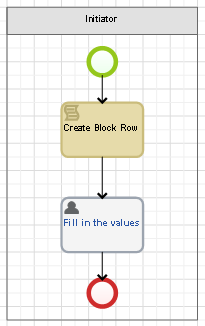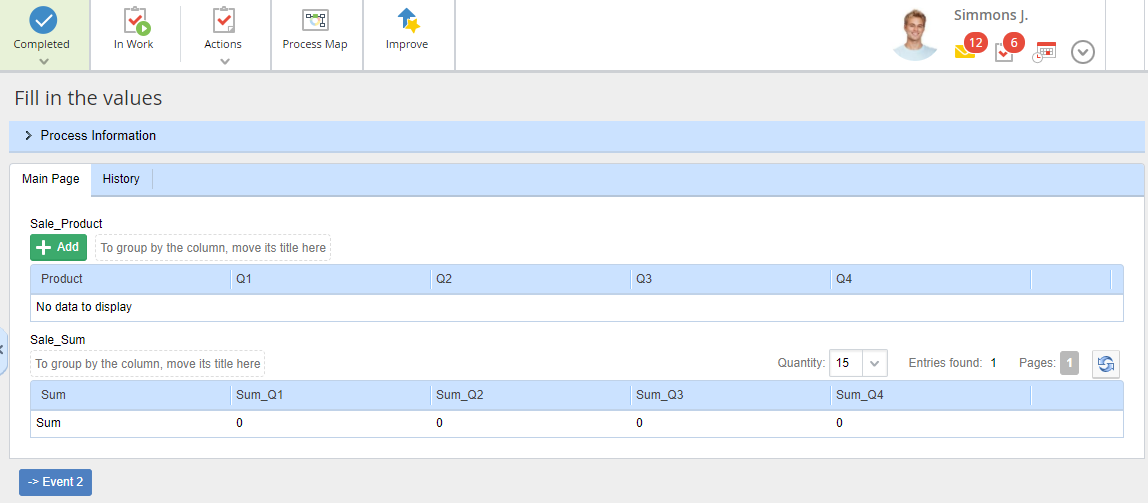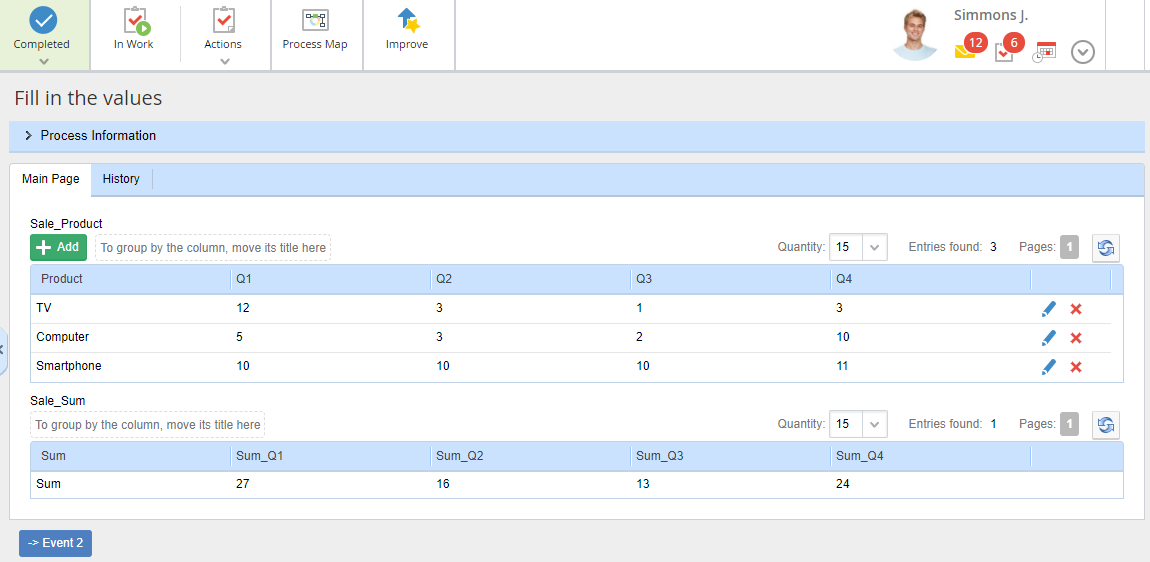# Dynamic forms for business process tasks: writing the result of operations with a block entries to another block

When using blocks in process tasks you may need to write the variable values from one block to the variables of another block. Let's take as an example writing the total of column values from one block to another.

In this example, we have two blocks:

1) Sale_Product block

The block contains the information on the amount (pcs.) of the company's product sold for each quarter.

Block properties:

Product – product, String type;

Q1 – product sales volume in the first quarter, Integer type;
Q2 – product sales volume in the second quarter, Integer type;
Q3 – product sales volume in the third quarter, Integer type;
Q4 – product sales volume in the fourth quarter, Integer type.

2) Sale_Sum block

The block contains the information on the sales volume of all the products in each quarter. The block and its properties are read-only.

Block properties:

Sum – String type;
Sum_Q1 – sales volume of all the products in the first quarter, Integer type;
Sum_Q2 – sales volume of all the products in the second quarter, Integer type;
Sum_Q3 – sales volume of all the products in the third quarter, Integer type;
Sum_Q4 – sales volume of all the products in the fourth quarter, Integer type.

Let's create a script that will automatically fill in the values of the second block when filling in the values of the first block. For this, use a standard on-change script, bound to the Sale_Product variable. This script will be executed when adding a new entry or saving the changes in an existing entry in the Sale_Product block. I.e. each time the Sale_Product block is changed, the values of the Sale_Sum block are recalculated.``using EleWise.ELMA.Model.Services;``

Initially, when opening a task, the Sale_Sum block will have no rows, therefore you need to create a row that will contain the sums:

``````public void Sum(Context context, EleWise.ELMA.Model.Views.FormViewBuilder<Context> form)
{
var newRow = InterfaceActivator.Create<P_Blok_Sale_Sum>(); //create a row in the Sale_Sum block
newRow.Sum_Q1 = 0; // set the "0" value to the cells
newRow.Sum_Q2 = 0;
newRow.Sum_Q3 = 0;
newRow.Sum_Q4 = 0;
newRow.Sum = "Sum"; //set the "Sum" value to the first cell
}``````

Now sum up the values of the first block Sale_Product and write the result to the second block Sale_Sum. To pass the values, use additional variables:

Total_Q1 – sales volume of all the products in the first quarter, Integer type;
Total_Q2 – sales volume of all the products in the second quarter, Integer type;
Total_Q3 – sales volume of all the products in the third quarter, Integer type;
Total_Q4 – sales volume of all the products in the fourth quarter, Integer type.

``````public void Sum_block(Context context, EleWise.ELMA.Model.Views.FormViewBuilder<Context> form)
{

context.Total_Q1 = 0; // set the "0" value to the context variables
context.Total_Q2 = 0;
context.Total_Q3 = 0;
context.Total_Q4 = 0;

foreach (var item in context. Sale_Product)
{
if(item.Q1 == null) // check if the cell value is filled in, if not, set the "0" value
item.Q1 = 0;
if(item.Q2 == null)
item.Q2 = 0;
if(item.Q3 == null)
item.Q3 = 0;
if(item.Q4 == null)
item.Q4 = 0;

context.Total_Q1 += item.Q1; //add the value of the Sale_Product block cell to the context variable
context.Total_Q2 += item.Q2;
context.Total_Q3 += item.Q3;
context.Total_Q4 += item.Q4;
}

foreach (var item in context.Sale_Sum.ToList())
{
item.Sum_Q1 = context.Total_Q1; //write the values of context variable to the Sale_Sum block cells
item.Sum_Q2 = context.Total_Q2;
item.Sum_Q3 = context.Total_Q3;
item.Sum_Q4 = context.Total_Q4;
}``````

Important
When working with block entries, DO NOT call the item.Save() method, since it can corrupt the database integrity.

You can find examples of scripts for blocks here.

If you create a simple process, you will get the following display in the web part.When opening the Fill in values task, a row of the Sale_Sum block is already created and it is assigned with initial values:After adding rows to the Sale_Product block, the values of the Sale_Sum block are recalculated automatically:Such an implementation may be useful when one user enters KPI values in a process, and then another user needs only the aggregated values on the task form.